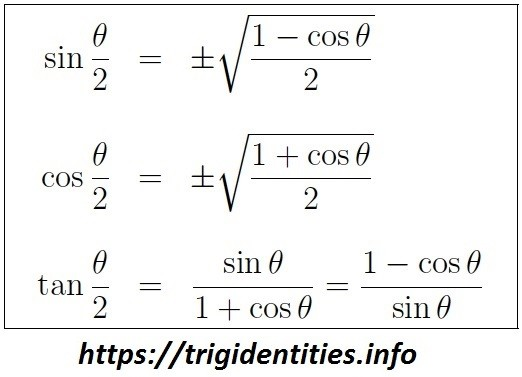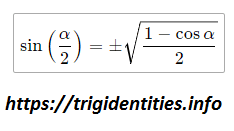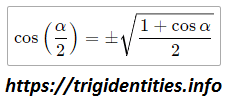# Trig Half-Angle Identities

Trig half angle identities or functions actually involved in those trigonometric functions which have half angles in them. The square root of the first 2 functions sine & cosine either negative or positive totally depends upon the existence of angle in a quadrant. Learn more about Trig Identities at trigidentities.info.Here comes the comprehensive table which depicts clearly the half-angle identities of all the basic trigonometric identities. Explore more about Inverse trig identities.

## Derivation of Trig Half-Angle Identities

Today we are going to derive following trig half-angle formulas.### Half Angle Formula – Sine

• cos 2θ = 1− 2sin2 θ

Now, if we let

• θ = α/2

then 2θ = α and our formula becomes:

• cosα=12 sin2(2α )

We now solve for

• Sin (α/2)
• 2 sin2(2α )=1−cosα
• sinˆ2 (2α) = 1 – cosα

Solving gives us the following sine of a half-angle identity:Now let talk about the positive or negative sign of Sin (α/2).

1. If the angle lies in the first quadrant then all positive means sine half angle identity will be positive.
2. And if it is in 3rd or 4th quadrant we will introduce a negative sign with the sine half angle identity.

### Half Angle Formula – Cosine

Simply by using a similar process, With the same substitutions, we did above. Now we have to substitute these values into the following Trig identity.

• cos 2θ = 2cos2 θ – 1

After substituting the values We

• Cosα = 2 cosˆ2(α/2)-1  —–(1)

Now you need to reverse the equation.

Reverse the equation:

• 2 cosˆ2(α/2)-1 = Cosα —–(2)

Add both eq (1) & (2)

• 2 cosˆ2(α/2) = 1+cosα

Divide by 2 on both sides

• cosˆ2(α/2) = 1+cosα/2

Taking square root on both sides we got cosine half angle formula nowlet’s talk about the positive or negative sign of Cosine (α/2).

• If the angle lies in first or 4th quadrant then Cosine(α/2) will be positive
• And if it is in 2nd or 3rd quadrant we will introduce a negative sign with the cosine half angle identity.